## 基數預估(cardinality estimate)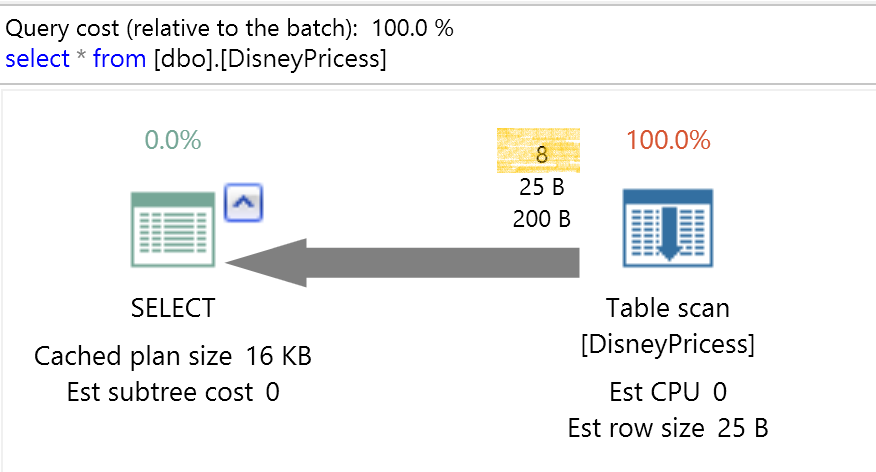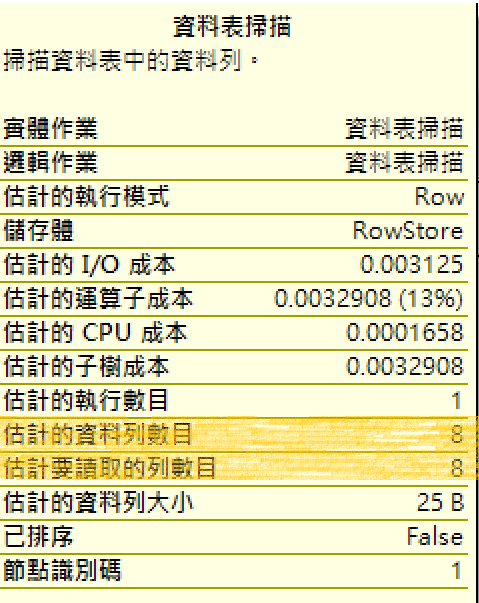## 測試環境

``````CREATE TABLE BANK
(C1 INT,C2 INT,C3 INT)
INSERT INTO BANK VALUES(1,2,3),(1,2,3),(1,2,3),(2,4,6),(2,4,6),(3,6,9)
SELECT * FROM BANK WHERE C1=1  AND C2=1
CREATE INDEX IX_BANK ON BANK(C1,C2,C3)
``````

## 看見統計值(Beyond Statistics)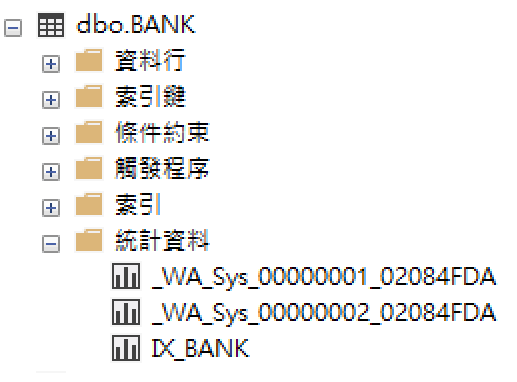• IX_BANK是索引欄位統計值項目。
• _WA_sys分別是兩個欄位的統計值項目。

### System view(sys.stats)

``````select * from sys.stats  s
where s.object_id = OBJECT_ID('BANK')``````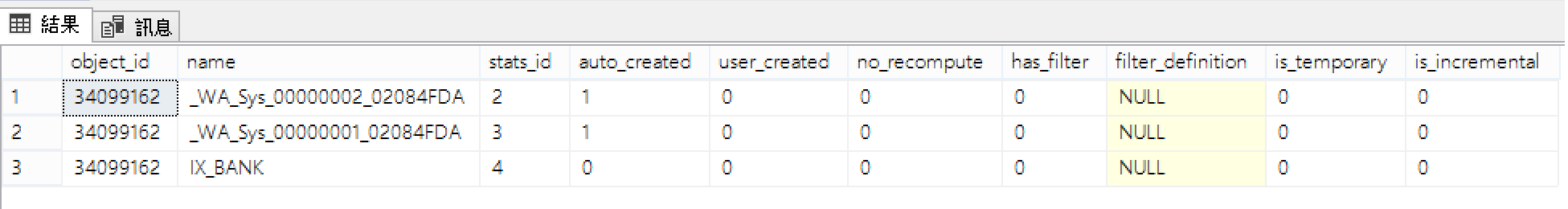### System Procedure(SP_Autostats)

``SP_Autostats 'BANK'``### DBCC Command(SHOW_STATISTICS)

``DBCC SHOW_STATISTICS('BANK','IX_BANK')``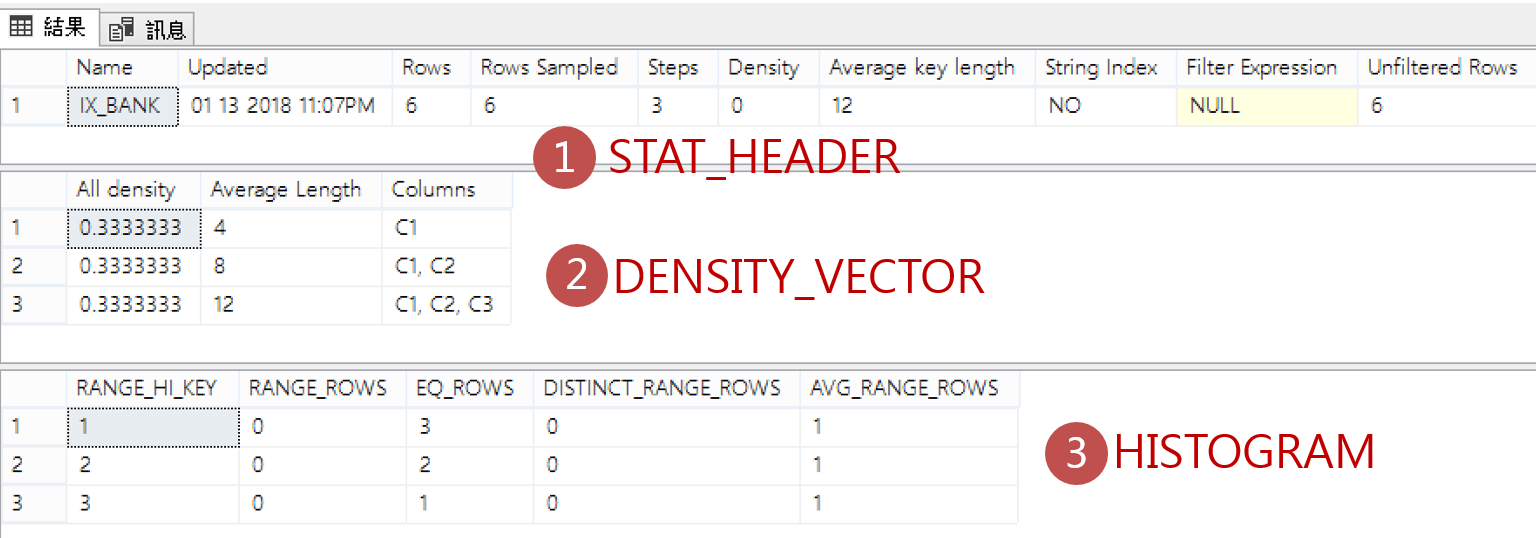## 長條圖(HISTOGRAM)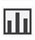就像我們在管理工具上看到的統計值圖示，每個統計值都會包含長條圖(histogram)，長條圖內容儲存欄位值的分佈(Distribution)，建立長條圖時，Query Optimizer會排序欄位值、計算相異數目，然後將結果彙總成最多200個連續的區間。

*只包含第一個欄位

SQL 2016 SP1之後多了一個DMV可以查詢，sys.dm_db_stats_histogram，效果等同DBCC SHOW_STATISTICS WITH HISTOGRAM

``````SELECT
OBJECT_NAME(s.object_id) AS TableName
,s.name
,COL_NAME(scol.object_id, scol.column_id) AS 'Column'
,hist.step_number
,hist.range_high_key
,hist.range_rows
,hist.equal_rows
,hist.distinct_range_rows
,hist.average_range_rows
FROM sys.stats AS s
JOIN sys.stats_columns AS scol (NOLOCK)
ON s.stats_id = scol.stats_id
AND s.object_id = scol.object_id
AND scol.stats_column_id = 1
CROSS APPLY sys.dm_db_stats_histogram(s.[object_id], s.stats_id) AS hist
WHERE s.object_id = OBJECT_ID('BANK')
ORDER BY s.name,hist.step_number,COL_NAME(scol.object_id, scol.column_id)
``````

DMV查詢結果: 列出BANK資料表每個統計值的長條圖資訊(包含欄位名稱、步驟次、區間間隔範圍、區間內的筆數、相異資料的筆數、平均筆數等)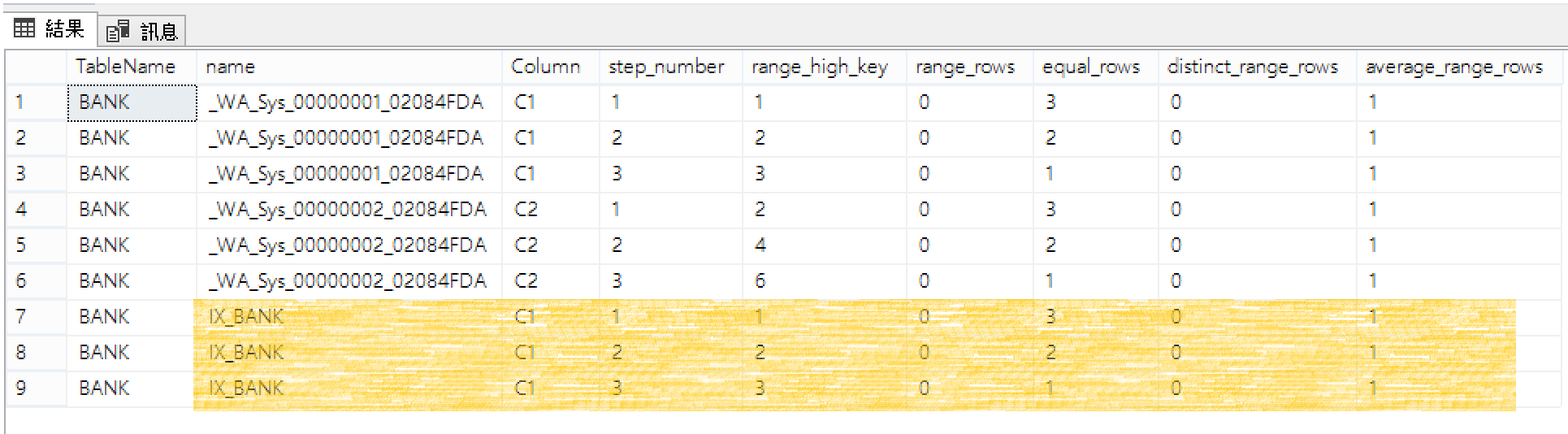DBCC SHOW_STATISTICS也能查出類似資訊，只差少了欄位名稱。

``DBCC SHOW_STATISTICS('BANK','IX_BANK') WITH HISTOGRAM``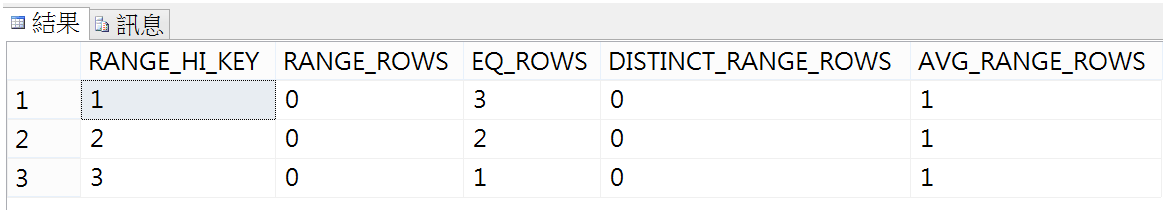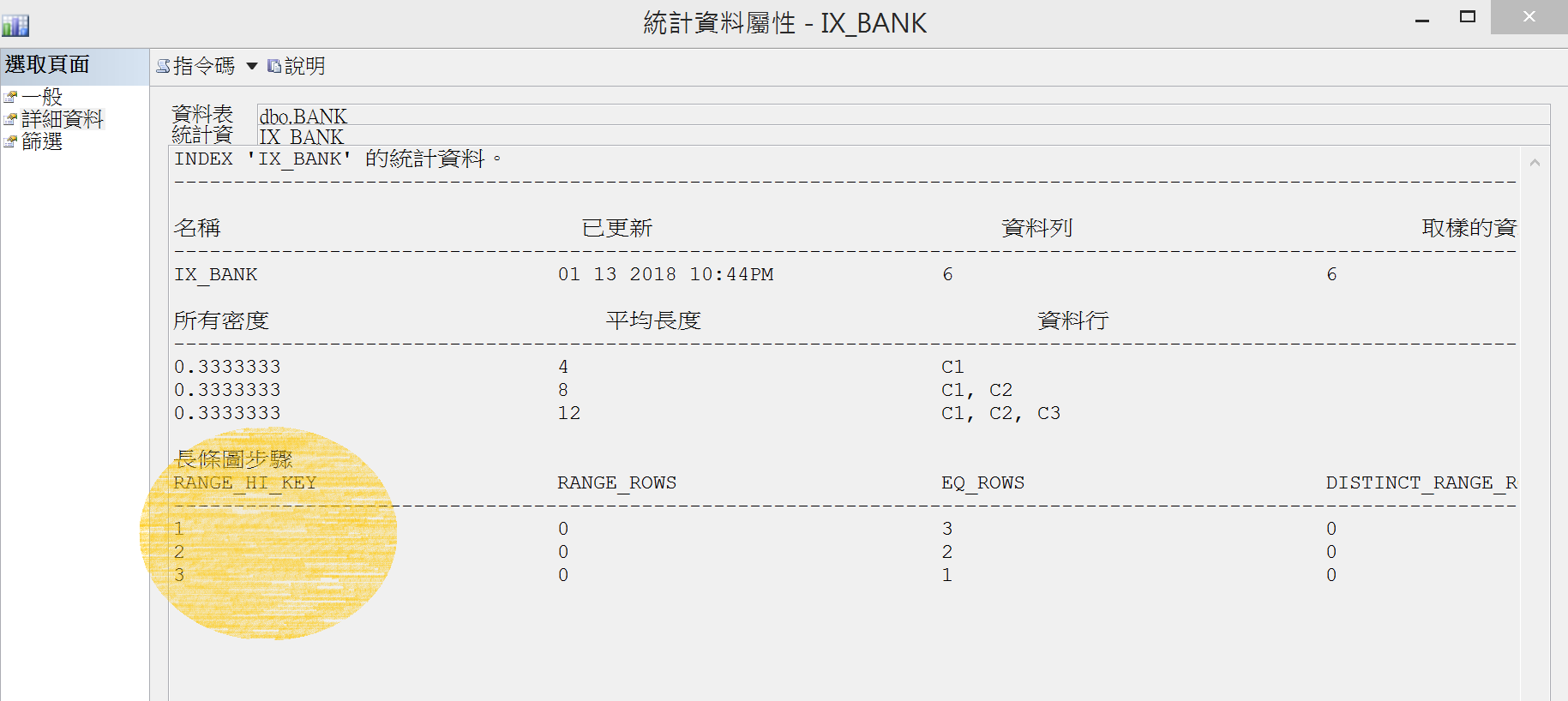## 密度(DENSITY):

(1,2,3),(1,2,3),(1,2,3),(2,4,6),(2,4,6),(3,6,9)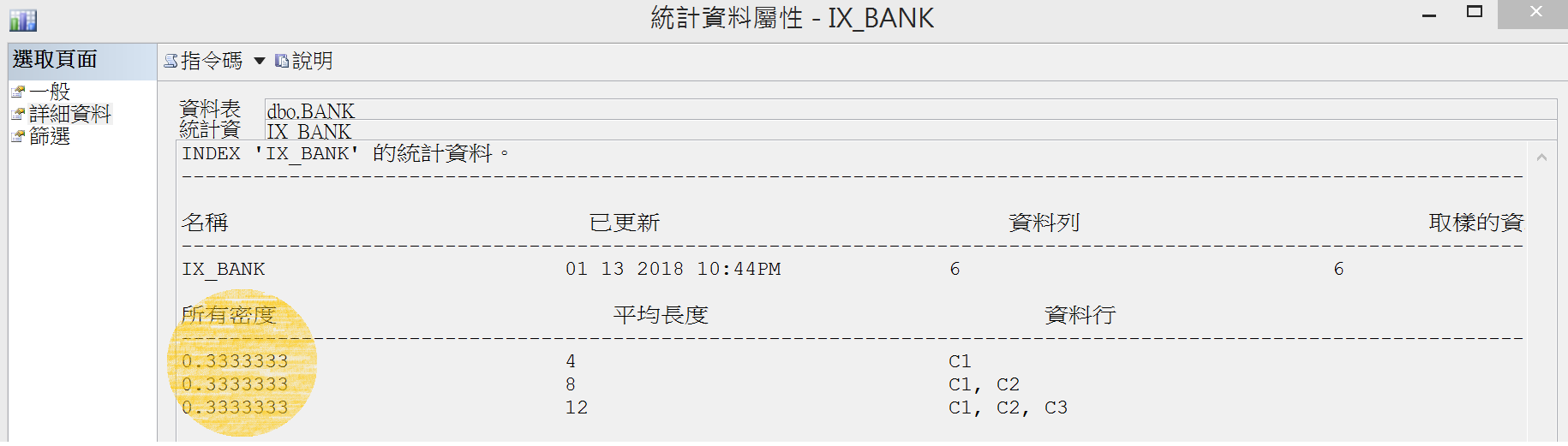DBCC SHOW_STATISTICS WITH DENSITY_VECTOR

``DBCC SHOW_STATISTICS('BANK','IX_BANK') WITH DENSITY_VECTOR``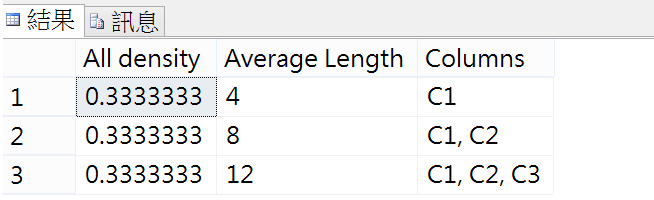### 觀察密度的變化

``````INSERT INTO BANK VALUES(1,99,99),(5,100,100)
UPDATE STATISTICS BANK
``````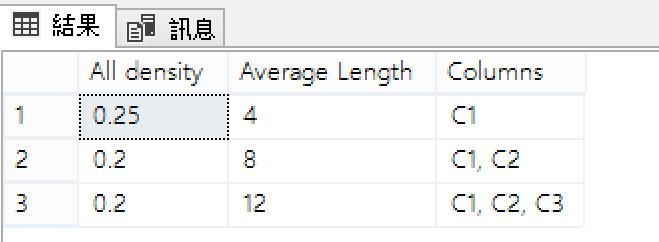``````Select [DistinctValue]=Count(Distinct C1),
[Density]=1/CAST(Count(Distinct C1) AS Float)
from BANK
GO
``````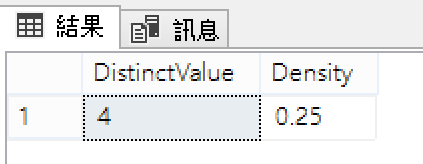``````SELECT C1,COUNT(*) FROM BANK
GROUP BY C1
``````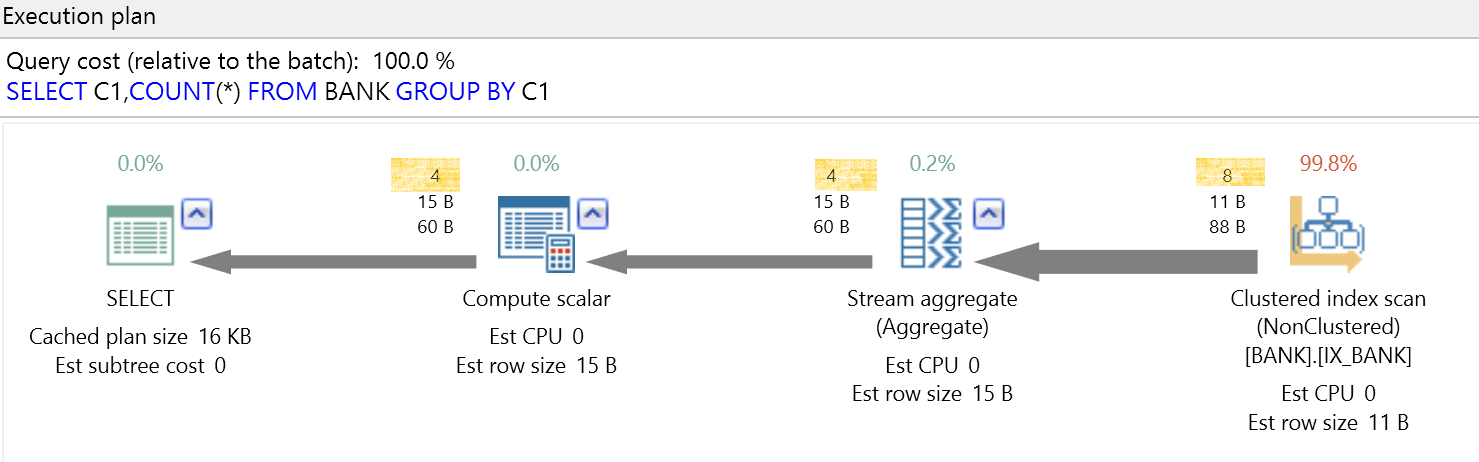2018/1/1 內湖瑞光路583巷，換新招牌的工程

## 參考

Docs sys.dm_db_stats_histogram

Docs system view-sys.stats

Docs system view-sys.stats_columns

Docs system-stored-procedures sp_statistics

Docs DBCC SHOW_STATISTICS